# RD Sharma Class 8 Solutions Chapter 13 Profits, Loss, Discount and Value Added Tax (VAT) Ex 13.3

In this chapter, we provide RD Sharma Class 8 Solutions Chapter 13 Profits, Loss, Discount and Value Added Tax (VAT) Ex 13.3 for English medium students, Which will very helpful for every student in their exams. Students can download the latest RD Sharma Class 8 Solutions Chapter 13 Profits, Loss, Discount and Value Added Tax (VAT) Ex 13.3 Maths pdf, free RD Sharma Class 8 Solutions Chapter 13 Profits, Loss, Discount and Value Added Tax (VAT) Ex 13.3 book pdf download. Now you will get step by step solution to each question.

### RD Sharma Solutions for Class 8 Chapter 13 Profits, Loss, Discount and Value Added Tax (VAT) Ex 13.3Download PDF

Question 1.
The list price of a refrigerator is Rs 9700. If a value added tax of 6% is to be charged on it, how much one has to pay to buy the refrigerator ?
Solution:
List price of refrigerator = Rs 9700
Rate of VAT = 6%
Amount of VAT = Rs. 9700×6100 = Rs 582
Total price to be paid = Rs 9700 + 582 = Rs 10282

Question 2.
Vikram bought a watch for Rs 825. If this amount includes 10% VAT on the list price, what was the list price of the watch ?
Solution:
Price of watch including VAT = Rs 825
Rate of VAT = 10%Question 3.
Aman bought a shirt for Rs 374.50 which includes 7% VAT. Find the list price of the shirt.
Solution:
Cost price of the shirt = Rs 374.50
Rate of VAT = 7%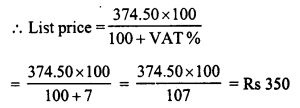Question 4.
Rani purchases a pair of shoes whose sale price is Rs 175. If he pays a VAT at the rate of 7%, how much amount does he pays as VAT. Find the net value of the pair of shoes.
Solution:
Sale price of a pair of shoes = Rs 175
Rate of VAT = 7%
Total VAT paid = Rs. 175×7100 = Rs 12.25
and total amount paid = Rs 175 + Rs 12.25 = Rs 187.25

Question 5.
Swarna paid Rs 20 as VAT on a pair of shoes worth Rs 250. Find the rate of VAT.
Solution:
Amount of VAT paid = Rs 20
Price of the pair of shoes = Rs 250
Rate of VAT = 20×100250 = 8%

Question 6.
Sarita buys goods worth Rs 5,500. She gets a rebate of 5% on it. After getting the rebate if VAT at the rate of 5% is charged, find the amount she will have to pay for the goods.
Solution:
Price of goods = Rs 5,500
Rate of rebate = 5%
Sales price after rebate= Rs 5,225
Rate of VAT = 5%
Amount of VATAmount to be paid after paying VAT = Rs 5,225 + 261.25 = Rs 5486.25

Question 7.
The cost of furniture inclusive of VAT is Rs 7,150. If the rate of VAT is 10%, find the original cost of the furniture.
Solution:
Cost of furniture including VAT = Rs 7,150
Rate of VAT = 10%
Original cost of furniture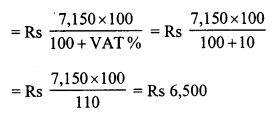Question 8.
A refrigerator is available for Rs 13,750 including VAT. If the rate of VAT is 10%, find the original cost of refrigerator.
Solution:
Cost of refrigerator including VAT = Rs 13,750
Rate of VAT = 10%
Actual price of refrigeratorQuestion 9.
A colour TV is available for Rs 13,440 inclusive of VAT. If the original cost of TV is Rs 12,000, find the rate of VAT.
Solution:
Cost of TV including VAT = Rs 13,440
Actual cost = Rs 12,000
Amount of VAT = Rs 13,440 – Rs 12,000 = Rs 1,440Question 10.
Reena goes to a shop to buy a radio, costing Rs 2,568. The rate of VAT is 7%. She tells the shopkeeper to reduce the price of the radio such that she has to pay Rs 2,568 inclusive of VAT. Find the reduction needed in the price of radio.
Solution:
Price of radio inclusive of VAT = Rs 2,568
Rate of VAT = 7%But the cost of radio in the beginning = Rs 2,568
Reduction = Rs 2568 – Rs 2,400 = Rs 168

Question 11.
Rajat goes to a departmental store and buys the following articles :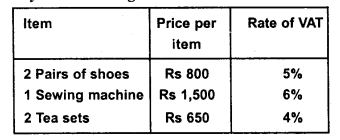Calculate the total amount he has to pay to the store.
Solution:
Price of 2 pairs of shoes @ Rs 800 = Rs800 x 2 = Rs 1,600
Rate of VAT = 5%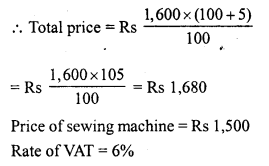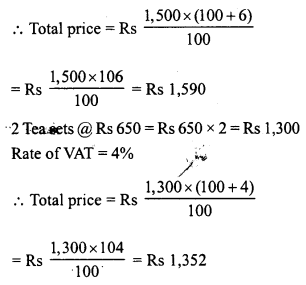Total amount to be paid = Rs 1,680 + Rs 1,590 + Rs 1,352 = Rs 4,622

Question 12.
Ajit buys a motorcycle for Rs 17,600 including value added tax. If the rate of VAT is 10%, what is the sale price of the motorcycle ?
Solution:
Cost price of motorcycle (including VAT) = Rs 17,600
Rate of VAT = 10%
Sale price of motorcycleQuestion 13.
Manoj buys a leather coat costing Rs 900 at Rs 990 after paying the VAT. Calculate the VAT charged on the cost.
Solution:
Cost price of coat = Rs 900
And sale price including VAT = Rs 990
Amount of VAT = Rs 990 – Rs 900 = Rs 90
Rate of VAT = 90×100900 = 10%

Question 14.
Rakesh goes to a departmental store and purchases the following articles:
(i) biscuits and bakery products costing Rs 50, VAT @ 5%.
(ii) medicines costing Rs 90. VAT @ 10%,
(iii) clothes costing Rs 400, VAT @ 1% and
(iv) cosmetics costing Rs 150, VAT @ 10% Calculate the total amount to be paid by Rakesh to the store.
Solution:
(i) Cost of biscuits and bakery product = Rs 50
VAT = 5%Total amount of the bill = Rs 52.50 + Rs 99 + Rs 404 + Rs 165 = Rs 720.50

Question 15.
Rajeeta purchased a set of cosmetics. She paid Rs 165 for it including VAT. If the rate of VAT is 10%, find the sale price of the set.
Solution:
Total price of set including VAT = Rs 165
Rate of VAT = 10%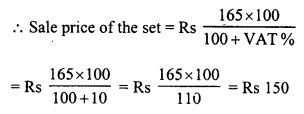Question 16.
Sunita purchases a bicycle for Rs 660. She has paid a VAT of 10%. Find the list price of bicycle.
Solution:
Cost price of bicycle including VAT = Rs. 660
Rate of VAT = 10%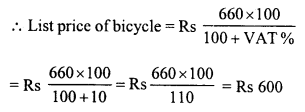Question 17.
The sales price of a television inclusive of VAT is Rs 13,500. If VAT is charged at the rate of 8% of the list price, find the list price of the television.
Solution:
Sale price of television including VAT = Rs 13,500
Rate of VAT = 8%Question 18.
Shikha purchased a car with a marked price of Rs 2,10,000 at a discount of 5%. If VAT is charged at the rate of 10%, find the amount Shikha had paid for purchasing the car.
Solution:
Marked price of car = Rs 2,10,000
Rate of discount = 5%Question 19.
Sharuti bought a set of cosmetic items for Rs 345 including 15% value added tax and a purse for Rs 110 including 10% VAT. What percent is the VAT charged on the whole transactions ?
Solution:
Cost price of set = Rs 345
VAT = 15%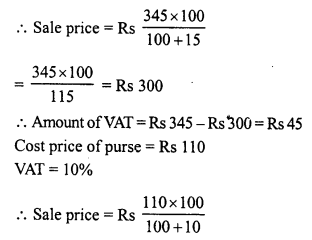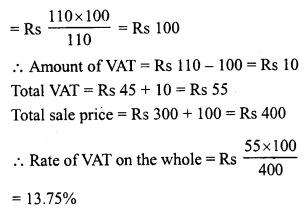Question 20.
List price of a cooler is Rs 2,563. The rate of VAT is 10%. The customer requests the shopkeeper to allow a discount in the price of the cooler to such an extent that the price remains Rs 2,563 inclusive of VAT. Find the discount in the price of the cooler.
Solution:
List price of cooler = Rs. 2,563
On request the price of cooler is paid Rs 2,563 including VAT
VAT = 10%Amount of discount = Rs 2,563 – Rs 2,330 = Rs 233

Question 21.
List price of a washing machine is Rs 9,000. If the dealer allows a discount of 5% on the cash payment, how much money will a customer pay to the dealer in cash, if the rate of VAT is 10%.
Solution:
List price of washing machine = Rs 9,000
Rate of discount = 5%
Amount after giving discount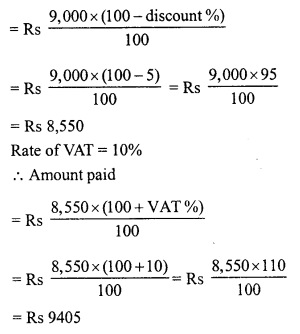All Chapter RD Sharma Solutions For Class 8 Maths

—————————————————————————–

All Subject NCERT Exemplar Problems Solutions For Class 8

All Subject NCERT Solutions For Class 8

*************************************************

I think you got complete solutions for this chapter. If You have any queries regarding this chapter, please comment on the below section our subject teacher will answer you. We tried our best to give complete solutions so you got good marks in your exam.

If these solutions have helped you, you can also share rdsharmasolutions.in to your friends.